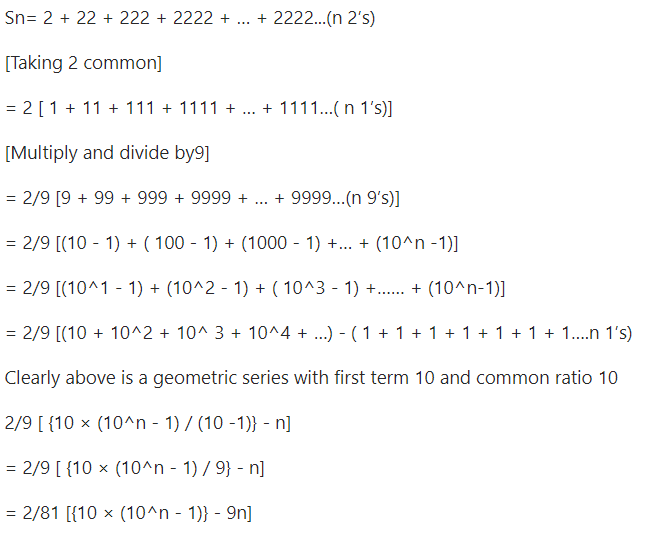Open in App
Not now

## Related Articles

• Write an Interview Experience
• Mathematical Algorithms

# Sum of the sequence 2, 22, 222, ………

• Difficulty Level : Medium
• Last Updated : 25 Jan, 2023

Find the sum of the following sequence : 2, 22, 222, ……… to n terms.
Examples :

```Input : 2
Output: 23.99868

Input : 3
Output: 245.98647```

A simple solution is to compute terms one by one and add to the result.
The above problem can be efficiently solved using the following formula:## C++14

 `// CPP program to find sum of series``// 2, 22, 222, ..``#include ``using` `namespace` `std;` `// function which return the``// the sum of series``float` `sumOfSeries(``int` `n)``{``    ``return` `0.02469 * (10*(``pow``(10, n) - 1)- (9 * n));``}` `// driver code``int` `main()``{``    ``int` `n = 3;``    ``cout << sumOfSeries(n);``    ``return` `0;``}`

## Java

 `// JAVA Code for Sum of the``// sequence 2, 22, 222,...``import` `java.util.*;` `class` `GFG {``    ` `    ``// function which return the``    ``// the sum of series``    ``static` `double` `sumOfSeries(``int` `n)``    ``{``        ``return` `0.02469` `* ((``10``*Math.pow(``10``, n)``                            ``- ``1``) - (``9` `* n));``    ``}``    ` `    ``/* Driver program */``    ``public` `static` `void` `main(String[] args)``    ``{``         ``int` `n = ``3``;``         ``System.out.println(sumOfSeries(n));``    ``}``}` `// This code is contributed by Arnav Kr. Mandal.`

## Python3

 `# Python3 code to find``# sum of series``# 2, 22, 222, ..``import` `math` `# function which return``# the sum of series``def` `sumOfSeries( n ):``    ``return` `0.02469` `*` `((``10``*``math.``pow``(``10``, n) ``-` `1` `)``-` `(``9` `*` `n))``    ` `# driver code``n ``=` `3``print``( sumOfSeries(n))` `# This code is contributed by "Sharad_Bhardwaj".`

## C#

 `// C# Code for Sum of the``// sequence 2, 22, 222,...``using` `System;` `class` `GFG {``    ` `    ``// Function which return the``    ``// the sum of series``    ``static` `double` `sumOfSeries(``int` `n)``    ``{``        ``return` `0.02469 * ((10*Math.Pow(10, n)``                           ``- 1) - (9 * n));``    ``}``    ` `    ``// Driver Code``    ``public` `static` `void` `Main()``    ``{``        ``int` `n = 3;``        ``Console.Write(sumOfSeries(n));``    ``}``}` `// This code is contributed by vt_m.`

## PHP

 ``

## Javascript

 ``

Output

`245.986`

Time complexity: O(log n) since using inbuilt power function.
Auxiliary Space: O(1)

My Personal Notes arrow_drop_up